# Excel COUNTIF and COUNTIFS with OR logic

The tutorial explains how to use Excel's COUNTIF and COUNTIFS functions to count cells with multiple OR conditions, e.g. if a cell contains X, Y or Z.

As everyone knows, Excel COUNTIF function is designed to count cells based on just one criterion while COUNTIFS evaluates multiple criteria with AND logic. But what if your task requires OR logic - when several conditions are provided, any one can match to be included in the count?

There are a few possible solutions to this task, and this tutorial will cover them all in full detail. The examples imply that you have a sound knowledge of the syntax and general uses of both functions. If not, you may want to begin with revising the basics:

Excel COUNTIF function - counts cells with one criteria.

Excel COUNTIFS function - counts cells with multiple AND criteria.

Now that everyone is on the same page, let's dive in:

## Count cells with OR conditions in Excel

This section covers the simplest scenario - counting cells that meet any (at least one) of the specified conditions.

### Formula 1. COUNTIF + COUNTIF

The easiest way to count cells that have one value or another (Countif a or b) is to write a regular COUNTIF formula to count each item individually, and then add the results:

COUNTIF(range, criterion1) + COUNTIF(range, criterion2)

As an example, let's find out how many cells in column A contain either "apples" or "bananas":

`=COUNTIF(A:A, "apples") + COUNTIF(A:A, "bananas")`

In real-life worksheets, it is a good practice to operate on ranges rather than entire columns for the formula to work faster. To spare the trouble of updating your formula every time the conditions change, type the items of interest in predefined cells, say F1 and G1, and reference those cells. For example:

`=COUNTIF(A2:A10, F1) + COUNTIF(A2:A10, G1)`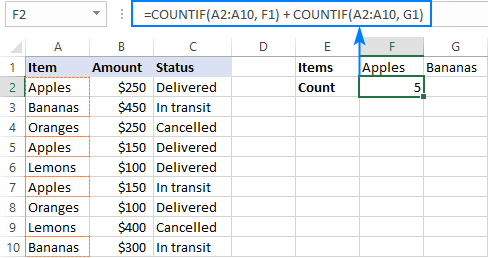This technique works fine for a couple of criteria, but adding three or more COUNTIF functions together would make the formula too cumbersome. In this case, you'd better stick with one of the following alternatives.

### Formula 2. COUNTIF with array constant

Here's a more compact version of the SUMIF with OR conditions formula in Excel:

SUM(COUNTIF(range, {criterion1, criterion2, criterion3, …}))

The formula is constructed in this way:

First, you package all the conditions in an array constant - individual items separated by commas and the array enclosed in curly braces like {"apples", "bananas', "lemons"}.

Then, you include the array constant in the criteria argument of a normal COUNTIF formula: COUNTIF(A2:A10, {"apples","bananas","lemons"})

Finally, warp the COUNTIF formula in the SUM function. It is necessary because COUNTIF will return 3 individual counts for "apples", "bananas" and "lemons", and you need to add those counts together.

Our complete formula goes as follows:

`=SUM(COUNTIF(A2:A10,{"apples","bananas","lemons"}))`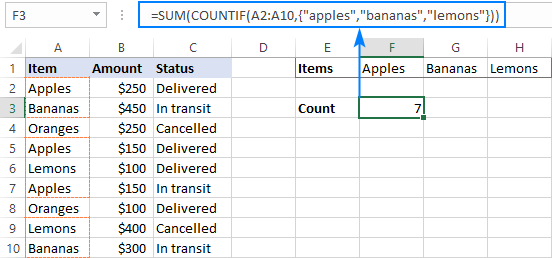If you'd rather supply your criteria as range references, you'll need to enter the formula with Ctrl + Shift + Enter to make it an array formula. For example:

`=SUM(COUNTIF(A2:A10,F1:H1))`

Please notice the curly braces in the screenshot below - it is the most evident indication of an array formula in Excel: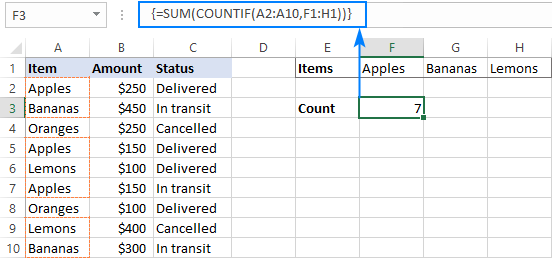### Formula 3. SUMPRODUCT

Another way to count cells with OR logic in Excel is to use the SUMPRODUCT function in this way:

SUMPRODUCT(1*(range ={criterion1, criterion2, criterion3, …}))

To better visualize the logic, this could also be written as:

SUMPRODUCT((range=criterion1) + (range=criterion2) + …)

The formula tests each cell in the range against each criterion and returns TRUE if the criterion is met, FALSE otherwise. As an intermediate result, you get a few arrays of TRUE and FALSE values (the number of arrays equals the number of your criteria). Then, the array elements in the same position are added together, i.e. the first elements in all the arrays, the second elements, and so on. The addition operation converts the logical values to numbers, so you end up with one array of 1's (one of the criteria matches) and 0's (none of the criteria matches). Because all the criteria are tested against the same cells, there is no way any other number could appear in the resulting array - only one initial array can have TRUE in a specific position, others will have FALSE. Finally, SUMPRODUCT adds up the elements of the resulting array, and you get the desired count.

The first formula works in a similar manner, with the difference that it returns one 2-dimentional array of TRUE and FALSE values, which you multiply by 1 to convert the logical values to 1 and 0, respectively.

Applied to our sample data set, the formulas take the following shape:

`=SUMPRODUCT(1*(A2:A10={"apples","bananas","lemons"}))`

Or

`=SUMPRODUCT((A2:A10="apples") + (A2:A10="bananas") + (A2:A10="lemons"))`

Replace the hardcoded array constant with a range reference, and you will get even a more elegant solution:

`=SUMPRODUCT(1*( A2:A10=F1:H1))`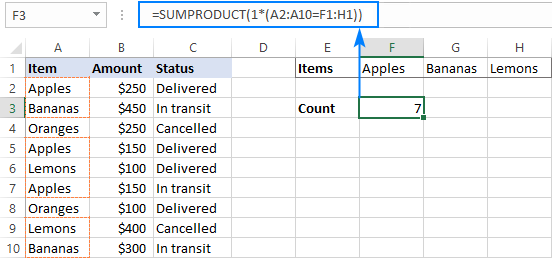Note. The SUMPRODUCT function is slower than COUNTIF, which is why this formula is best to be used on relatively small data sets.

## Count cells with OR as well as AND logic

When working with large data sets that have multi-level and cross-level relations between elements, chances are that you will need to count cells with OR and AND conditions at a time.

As an example, let's get a count of "apples", "bananas" and "lemons" that are "delivered". How do we do that? For starters, let's translate our conditions into Excel's language:

• Column A: "apples" or "bananas" or "lemons"
• Column C: "delivered"

Looking from another angle, we need to count rows with "apples and delivered" OR "bananas and delivered" OR "lemons and delivered". Put this way, the task boils down to counting cells with 3 OR conditions - exactly what we did in the previous section! The only difference is that you'll utilize COUNTIFS instead of COUNTIF to evaluate the AND criterion within each OR condition.

### Formula 1. COUNTIFS + COUNTIFS

It is the longest formula, which is the easiest to write :)

`=COUNTIFS(A2:A10, "apples", C2:C10, "delivered") + COUNTIFS(A2:A10, "bananas", C2:C10, "delivered")) + COUNTIFS(A2:A10, "lemons", C2:C10, "delivered"))`

The screenshot below shows the same formula with cells references:

`=COUNTIFS(A2:A10, K1, C2:C10, K2) + COUNTIFS(A2:A10, L1, C2:C10, K2) + COUNTIFS(A2:A10, M1,C2:C10, K2)`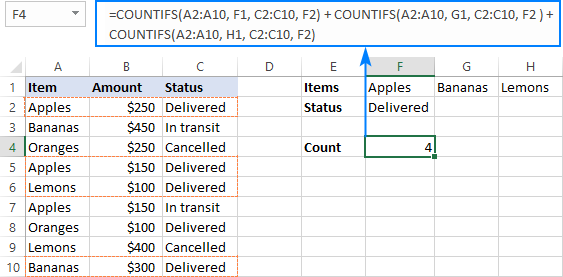### Formula 2. COUNTIFS with array constant

A more compact COUNTIFS formula with AND/OR logic can be created by packaging OR criteria in an array constant:

`=SUM(COUNTIFS(A2:A10, {"apples","bananas","lemons"}, C2:C10, "delivered"))`

When using a range reference for the criteria, you need an array formula, completed by pressing Ctrl + Shift + Enter:

`=SUM(COUNTIFS(A2:A10,F1:H1,C2:C10,F2))`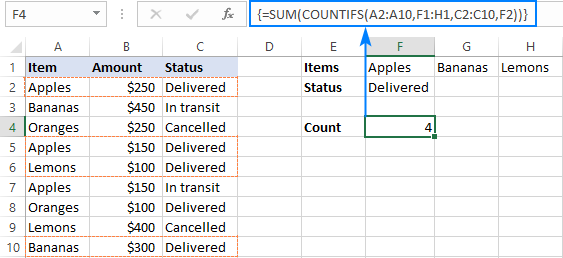Tip. If needed, you are free to use wildcards in the criteria of any formulas discussed above. For example, to count all sorts of bananas such as "green bananas" or "goldfinger bananas" you can use this formula:

`=SUM(COUNTIFS(A2:A10, {"apples","*bananas*","lemons"}, C2:C10, "delivered"))`

In a similar manner, you can build a formula to count cells based on other criteria types. For example, to get a count of "apples" or "bananas" or "lemons" that are "delivered" and the amount is greater than 200, add one more criteria range/criteria pair to COUNTIFS:

`=SUM(COUNTIFS(A2:A10, {"apples","*bananas*","lemons"}, C2:C10, "delivered", B2:B10, ">200"))`

Or, use this array formula (entered via Ctrl + Shift + Enter):

`=SUM(COUNTIFS(A2:A10,F1:H1,C2:C10,F2, B2:B10, ">"&F3))`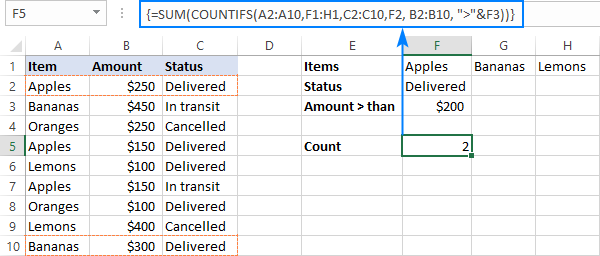## Count cells with multiple OR conditions

In the previous example, you have learned how to test one set of OR conditions. But what if you have two or more sets and you are looking to get a total of all possible OR relations?

Depending on how many conditions you need to handle, you can use either COUNTIFS with an array constant or SUMPRODUCT with ISNUMBER MATCH. The former is relatively easy to build, but it is limited to only 2 sets of OR conditions. The latter can evaluate any number of conditions (a reasonable number, of course, given Excel's limit to 255 arguments and 8192 characters to the total formula length), but it may take some effort to grasp the formula's logic.

### Count cells with 2 sets of OR conditions

When dealing with only two sets of OR criteria, just add one more array constant to the COUNTIFS formula discussed above.

For the formula to work, one minute but critical change is needed: use a horizontal array (elements separated by commas) for one criteria set and vertical array (elements separated by semicolons) for the other. This tells Excel to "pair" or "cross-calculate" the elements in the two arrays, and return a two-dimensional array of the results.

As an example, let's count "apples", "bananas" or "lemons" that are either "delivered" or "in transit":

`=SUM(COUNTIFS(A2:A10, {"apples", "bananas", "lemons"}, B2:B10, {"delivered"; "in transit"}))`

Please note the semicolon in the second array constant: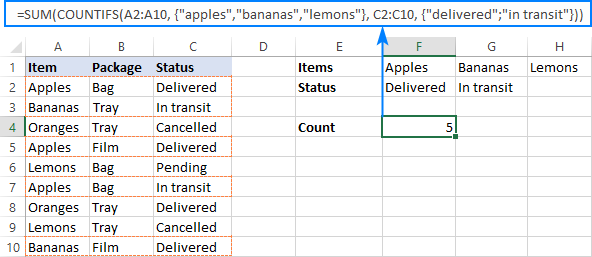Because Excel is a 2-dimentional program, it is not possible to construct a 3-dimentional or 4-dimentuional array, and therefore this formula only works for two sets of OR criteria. To count with more criteria, you will have to switch to a more complex SUMPRODUCT formula explained in the next example.

### Count cells with multiple sets of OR conditions

To count cells with more than two sets of OR criteria, use the SUMPRODUCT function together with ISNUMBER MATCH.

For example, let's get a count of "apples", "bananas" or "lemons" that are either "delivered" or "in transit" and are packaged in either "bag" or "tray":

```=SUMPRODUCT(ISNUMBER(MATCH(A2:A10,{"apples","bananas","lemons"},0))* ISNUMBER(MATCH(B2:B10,{"bag","tray"},0))* ISNUMBER(MATCH(C2:C10,{"delivered","in transit"},0))) ```

In the heart of the formula, the MATCH function checks the criteria by comparing each cell in the specified range with the corresponding array constant. If the match is found, it returns a relative position of the value if the array, N/A otherwise. ISNUMBER converts these values to TRUE and FALSE, which equate to 1 and 0, respectively. SUMPRODUCT takes it from there, and multiplies the arrays' elements. Because multiplying by zero gives zero, only the cells that have 1 in all the arrays survive and get summed.

Th screenshot below shows the result: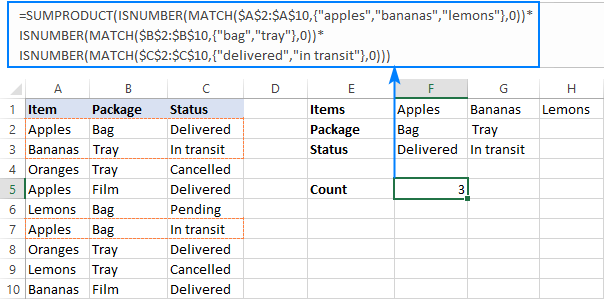This is how you use the COUNTIF and COUNTIFS functions in Excel to count cells with multiple AND as well as OR conditions. To have a closer look at the formulas discussed in this tutorial, you are welcome to download our sample workbook below. I thank you for reading and hope to see you on our blog next week!

## Practice workbook

Excel COUNTIF with OR conditions - examples (.xlsx file)

## You may also be interested in

1. Good morning! You all are so incredibly helpful, I just hope I can describe my issue well enough for you to understand! I have a table that looks like this:
Virtual Virtual Virtual
Onsite Onsite
OTT OTT
Jon X X X
Dan X X X
Rob X X X
Tim X X X

I need to count the number of times each person is either Onsite or OTT. The results I should get are:
Jon 2
Dan 1
Rob 0
Tim 3

However, when I try to use normal COUNTIFS it counts Jon and Tim twice because column C has both Onsite AND OTT. I need it to count Onsite OR OTT. I tried to use the OR logic you outlined... =SUM(COUNTIFS(\$B\$1:\$G\$3,{"Onsite","OTT"},B4:G4,"X")) but that doesn't work with multiple rows. How can I get this to calculate correctly? I feel like I'm so close...

• Okay, so multiple spaces get stripped out... Let's try with dots...
..........Virtual................Virtual................Virtual
........................Onsite...........................................Onsite
........................OTT....................OTT
Jon......................X.........................X.............X
Dan.........X.......................X......................................X
Rob.........X.......................X...........................X
Tim.....................X..........................X........................X

2. I am working on a spreadsheet with Start Date, Resolve Date, and Status. I want to look at the amount of work being done in the current month. For example, if I'd like to look at the number of things completed in August, I could count everything initiated in August that has a complete status PLUS everything resolved in August with a complete status. BUT, the problem is that some things can be initiated in August and Resolved in August, which would cause the same issue to be double counted.

My current formula for counting total complete based on start date is
=COUNTIFS(StartDate,">="&EOMONTH(TODAY(),-1)+1,StartDate,"="&EOMONTH(TODAY(),-1)+1,Resolved,"<"&EOMONTH(TODAY(),0)+1,Status,"Completed")

I'm not quite sure how to combine these into one formula and prevent the double counting of items that are completed with a start and resolve date in the same month. Any help would be greatly appreciated!!!

• I typed in two formulas, but it looks like it somehow got combined into two?? They should be as follows:

Based on start date:
=COUNTIFS(StartDate,">="&EOMONTH(TODAY(),-1)+1,StartDate,"="&EOMONTH(TODAY(),-1)+1,Resolved,"<"&EOMONTH(TODAY(),0)+1,Status,"Completed")

• I don't know why this keeps happening... There are two formulas that I'm typing in, with a new paragraph between them. My paragraph keeps getting deleted and the formulas partially combined.

• Hello!
I can't check the formula that contains unique references to your workbook worksheets. Count the records that meet the first condition, add the records that meet the second condition, and subtract the records that meet both conditions.

=COUNTIFS(StartDate,”>= ”&EOMONTH(TODAY(),-1)+1,Status,"Completed") + COUNTIFS(Resolved,”<"&EOMONTH(TODAY(),0)+1,Status,"Completed") - COUNTIFS(StartDate,”>=”&EOMONTH(TODAY(),-1)+1, Resolved,”<"&EOMONTH(TODAY(),0)+1,Status,"Completed")

3. Hi,

I am trying to create a column that will provide me a subset of orders that need to be reconciled between two ERP systems. However, there are three different types of ways that one system will recognize an order.

I am hoping that this column will catch IF it exists in one system under one reference, but right now there are cases where an order can be recognized by all three references, just depends on user input.

Trying to acheive a formula that will count Order Ref 1 OR Order Ref 2 or Order Ref 3. If the system recognizes it as Order Ref 1 / Order Ref 2 I only want it to count once towards the total subset.

Current formula: =COUNTIF('TMS DATA'!A:A, Table2[[#All],[Order '#]])+COUNTIF('TMS DATA'!A:A,Table2[[#All],[Shipment'#]])+COUNTIF('TMS DATA'!A:A,Table2[[#All],[Order ID]])

Can you help me with the OR part that I am missing? I feel like I am just counting them all.

• Hi!
It is very difficult to understand a formula that contains unique references to your workbook worksheets. Unfortunately, without seeing your data it is difficult to give you any advice. Please provide me with an example of the source data and the expected result.

4. Can anyone help with the formula?

I have 10 drivers and 10 vehicles, Each day wages of driving individual vehicle is different. I.e Vehicle A wages 500, vehicle B wages 1000 and Vehicle C Wages 1500. Now Driver A had drove those vehicles in term of 15 days. How would I calculate this with the formula.

5. Hello and thank you for you dedication to helping people!

I believe there is an issue with the AND/OR logic part of the article in that the formula generates "false positives" which must be subtracted to get a correct answer.

LIST A LIST B LIST C LIST D
TRUE TRUE TRUE TRUE
TRUE TRUE TRUE FALSE
TRUE TRUE FALSE TRUE
TRUE TRUE FALSE FALSE
TRUE TRUE TRUE TRUE
TRUE TRUE TRUE FALSE
TRUE FALSE FALSE TRUE
TRUE FALSE FALSE FALSE
TRUE FALSE TRUE TRUE
TRUE FALSE TRUE FALSE
TRUE FALSE FALSE TRUE
TRUE FALSE FALSE FALSE

For instance, if (A and B) or C or D are true in the above data the result should be 5 (not 7) as below:

=COUNTIFS(LIST_A,TRUE,LIST_B,TRUE,LIST_C,TRUE)+COUNTIFS(LIST_A,TRUE,LIST_B,TRUE,LIST_D,TRUE)-COUNTIFS(LIST_A,TRUE,LIST_B,TRUE,LIST_C,TRUE,LIST_D,TRUE)

Here's the rub. If I have additional columns (not shown and also not actual data) and need to find (A and B) or C or D or E or F or G or H or I or J or K or L in a list of 100,000 items (peak season), is an elegant solution possible?

Thanks and I do appreciate your work!

• Hi!
Your formula returns 5. But if I understood your conditions correctly, the result should be 3.
If I understand your task correctly, the following formula should work for you:

=SUM(--((A2:A13+B2:B13+(C2:C13+D2:D13=1)=3)))

• Thank you for the prompt response. Unfortunately I did not clearly state the full nature of the problem.

Data is not "true" or "false" but could be text, currency, value, date, blank, etc.
Each line is counted only once based on conditions further explained as follows:
List A and List B must both be "true" for a line to be counted.
Lists E through L must have at least one condition "true" for a line to be counted.
Each line may only be counted as one instance.
So lines 1, 2, 3, 5, 6 should be the only lines counted for a total of 5 lines meeting the conditions.

Helper columns discouraged because of the size of the data.

I perceive this as Wizardry at this point.

• Hi!

=SUM(--(((A2:A13=TRUE)*(B2:B13=TRUE)*100+(C2:C13=TRUE)+(D2:D13=TRUE)) > 100))

• Sir, that is a brilliant and imaginative solution! This is a truly remarkable method for implementing complex AND/OR conditions in Excel. Thank you and may the formulas be always in your favor.

6. Hello. The "count cells with multiple sets of OR conditions" formula described above was extremely helpful for me. Thank you!

Let's say there's a "Date_Shipped" column in D:D.

How can I find {apples, bananas, lemons}, {bag,tray}, {delivered, in transit} ***where Date_Shipped > mm/dd/yyyy and Date_Shipped < mm/dd/yyyy***

This is the formula from the example above I'm using and just need to figure out how to incorporate the date part:

=SUMPRODUCT(ISNUMBER(MATCH(A2:A10,{"apples","bananas","lemons"},0))*

ISNUMBER(MATCH(B2:B10,{"bag","tray"},0))*

ISNUMBER(MATCH(C2:C10,{"delivered","in transit"},0)))

• Hello!
If you write dates in column B, then you can compare them with the desired date using the DATE function.

=SUMPRODUCT(ISNUMBER(MATCH(\$A\$2:\$A\$10, {"apples","bananas","lemons"},0))*ISNUMBER(MATCH(\$B\$2:\$B\$10, {"bag","tray"},0))*ISNUMBER(MATCH(\$C\$2:\$C\$10, {"delivered","in transit"},0))*(--(\$D\$2:\$D\$10 > DATE(2022,2,1))*(--(\$D\$2:\$D\$10 < DATE(2022,3,1)))))

Hope this is what you need.

• Thank you! I was not familiar with the double minus (--). This is exactly what I needed and the formula works like a charm. CHEERS!

7. I have a worksheet that I am working for work. Basically, I would like to count how many people visit the park (column A) in both year 2020 and 2021(column B). Column B only shows either 2020 or 2021.

This formula: =SUM(COUNTIFS(Table2[List YR], {"2020","2021"}, Table2[COUNTY], A2))
- provides number of people that visit on 2020 and number of people that visit on 2021, not the number of people that visit both year.

This formula: =COUNTIFS(SPEC!A:A,A2,SPEC!B:B,2020,SPEC!B:B,2021)
- provides 0

• Hello!
Add column C to the table with the formula

=IF(COUNTIFS(\$A\$2:\$A\$10,A2,\$B\$2:\$B\$10,2021)>0,2021,"")

Count the number of people using COUNTIFS:

=COUNTIFS(B2:B10,2020,C2:C10,2021)

8. If a student gets mark less than 16 or his average be less than 50 he must be in group D. Please write a formula for it

• Hi!
Please check out this article to learn how to write an IF OR statement in Excel to check for various "this OR that" conditions.

9. Hello!
My husband is trying to create a spreadsheet for work and cannot seem to find a formula that works.
He is trying to use the COUNTIFS function to count all cells in a range that are within separate number ranges.

He needs the cells to be counted once if they are below 517, or between 525 and 896, or above 905. Every formula we have tried has not worked. It either does not count the cells, or counts them more than once.

This is an example of one of the formulas we have tried:

=COUNTIFS(C3:I4,">=0","525","905")

I know this is not right, but I have scoured the internet but have not found a solution.

Thank you!

10. Hey, can you help me with a google sheet formula?

=SUM(COUNTIF('Signed Case'!C:C,{"Barry","Maya"}))

It seems that Google Sheet can only count the total number of "Barry" but NOT BOTH "Barry" and "Maya", is there a way to fix this?

• =SUM(COUNTIF('Signed Case'!C:C,{"Barry","Maya"})) - Wrong Formula

Correct one

=SUM(COUNTIFS('Signed Case'!C:C,{"Barry","Maya"})) -than press Ctrl+shift+Enter

11. Hello!

I have been trying to count the number of LGBTQ+ from an organization. However, the code

SUM(COUNTIF(range, {criterion1, criterion2, criterion3, …}))

does not seem to work. Here is a sample of my work. I hope you can visualize it.

=SUM(COUNTIFS(E169:E209,{"P","Bi"}))

Note: COUNTIFS or COUNTIF, still does not work. It does not count the "Bi"

12. I am trying to count Yes and No in 1 cell but from multiple columns and then create a score in another column. So if I got 3 "yes" and 1 "no" the score would be 3. This what I got:
=SUM(COUNTIF(U3,N3,X3,{"Yes",1})(U3,N3,X3,{"No",0}))
But it did occur to me that I don't need to count the "No" so could I shorten it thus?
=SUM(COUNTIF(U3,N3,X3,{"Yes",1})

I'm getting errors saying I'm entering too many arguments and it doesn't work. Help!

13. Hello!

Trying to find a solution for nested conditions with no success so far. Please see below:

Spreadsheet is looking to see if a range of cells (D:32:D35) is answered "Yes", If 1-2 cells in this range are answered "Yes", then cell L:31 will be assigned a value of .5. However if greater than 2 cells in the range answer "Yes". then L:31 will need to be 1.

Any tips? Thank you!

• Hi!

=IF(COUNTIF(D2:D35,"Yes")<3,0.5,1)

14. Since, after multiple attempts, I can't seem to get my formula to show correctly, can I email it? It shows fine, until I hit Send and then half of it is missing.

• We apologize for this nasty behavior. Our blog engine often mangles formulas containing "less than" and "greater than" operators, and we can do nothing about it. You can email your formula to support@ablebits.com Att: Alexander Trifuntov

Again, our apologies for the inconvenience.

• Thank you for your emailed response, which worked.

15. SUMPRODUCT(--(AND('Source Sheet'!\$D:D>=A3,'Source Sheet'!\$D:D0,1,0))

• Aaarrrrgghhhh!!!!!

16. I don't understand why the formulae are being truncated.

17. Breaking it up, will see if that works

=SUMPRODUCT(--(AND('Source Sheet'!\$D:D>=A3,'Source Sheet'!\$D:D0,1,0))

18. I want to count, only once, those values that match ONE of a range of three criteria. Column A contains dates and multiple rows can have the same date. Columns B, C or D contain only "Y" or "N". I want to count, for each date, only those rows where a "Y" is in any one of B, C or D. If there is an N in all three, it is not counted. For example:

01/01/2021 Y N N
01/01/2021 N N Y
01/01/2021 N N N
01/01/2021 Y Y Y
02/01/2021 Y Y Y

The count against 01/01/2021 should return 3 above, because there is at least one Y in cols B, C or D on three of the four rows that match that date (where there is more than one, it should only be counted once).

No variation of SUMPRODUCT, COUNTIFS etc I have come up with gives me the right number. Can you help please?

• Hello!
Please check the formula below, it should work for you:

=SUMPRODUCT(--(\$A\$1:\$A\$10=A1),IF((\$B\$1:\$B\$10="Y")+(\$C\$1:\$C\$10="Y")+(\$D\$1:\$D\$10="Y")>0,1,0))

I hope this will help

• It does for individual dates, thank you. I also have a monthly summary sheet. In this one, months (eg Jan-21, Feb 21 etc are in column A of the summary sheet, to be checked against individual dates on column D of the source worksheet and the data to be checked for a single Y are in columns E to G of the source worksheet. I have attempted to adapt the formula you gave but this returns a VALUE error. I am guessing it is a simple missed comma or bracket, or is it the AND throwing it out?

=SUMPRODUCT(--(AND('Source Sheet'!\$D:D>=A3,'Source Sheet'!\$D:D0,1,0))

This is attempting to count, for all dates between 1st and last date of the month in column A of the target sheet, where there is a Y on each row in any one of columns E to G in the source sheet,

For example, if A3 on the target sheet were Mar-21, it would retrieve all values where the date is greater than or equal to 1st March 2021 and less than or equal to 31st March 2021 and, for the rows between those dates in the source sheet, count how many of those rows have at least one Y between cells E and G and output that number to the cell containing the above formula in the target sheet. Note, I have ruled out using just month numbers as the source sheet contains more than one year of dates.

• Some of the formula was missing in my last comment. Trying again

=SUMPRODUCT(--(AND('Source Sheet'!\$D:D>=A3,'Source Sheet'!\$D:D0,1,0))

19. I am creating an spreadsheet for our Oncology dept which has the following sheets say 3 March, 4 March, 5 March etc and then a summary page. Each days sheet contains the Patient ID as well as what type, namely chemo, New File, Others etc. In the summary page I need to show a summary count with each of the category (Chemo,New Fileetc.) under a day column like this

05 March 2021 06 March 2021 07 March 2021

Morning Session - New File 1 #VALUE!
Morning Session - Chemo 2
Morning Session - Old 1
Morning Session - Others 0

***** 4
The COUNTIFS formula works when I dont have to evaluate the date to the current date which I have given as a heading and then in a cell in the days (6th March sheet). That throws an error. This is the formula I have used
=COUNTIFS('6th March'!E5:E1335,"Chemo", ',Summary!C2,'6th March'!C2)
Where the second criteria is the date I am trying to collate.

Any help will be much appreciated.

• Hello!
Unfortunately, without seeing your data it is impossible to give you advice.
I'm sorry, it is not very clear what result you want to get. Could you please describe your task in more detail and send us a small sample workbook with the source data and expected result to support@ablebits.com? Please shorten your tables to 10-20 rows/columns and include the link to your blog comment.

20. Hi,

I am currently working on a formula to track 3 different types of orders and their status. In the workbook I have the order type as well as the due date and completion date in separate columns. I also created 2 columns to calculate IF statements to determine if 1) the order is "overdue" (completion date>due date) and 2) if the completion date is "blank" to mark it as outstanding. Currently I have the formula set to count any of the specific order type that is marked as overdue or as outstanding (Formula:=COUNTIFS('Due Dates'!\$A:\$A, T18,'Due Dates'!I:I, "OVERDUE")+COUNTIFS('Due Dates'!\$A:\$A, T18,'Due Dates'!J:J, "OUTSTANDING"); however, I want to narrow it down further so that only pulls the overdue+outstanding count from a specific due date instead of from everything. What function should I use to go about this? Thank you!

• Hello!
If I understand your problem correctly, one more condition needs to be added to each of the COUNTIFS formulas. Like that:

=COUNTIFS('Due Dates'!\$A:\$A, T18,'Due Dates'!I:I, "OVERDUE",'Due Dates'!B:B,">"&T19 )+COUNTIFS('Due Dates'!\$A:\$A, T18,'Due Dates'!J:J, "OUTSTANDING",'Due Dates'!B:B,">"&T19)

where T19 is the date to count.
Hope this is what you need.

21. Hi,

Help me with the count function for a number and a text on the same range. For example, I want to count cells based on attendance, if it is Y, it should be considered, if I update as 1 or 30, it should also be counted for all the data

• Hi,
If I understand your task correctly, the following formula should work for you:

=SUM(COUNTIF(A1:A10,{1,30,"Y"}))

I hope this will help

22. Need help. I need to an item in a separate excel spreadsheet to be counted based on two conditions in a worksheet. I need it to count the item once if the Part No of the item (for example is 01667-1" and the order number column is populated with an all numerical number (for examples " 1234" or #1556"). The numerical number is unique except for the first number so I tried using a wild card. Oh and one more thing the numerical column (G:G) may have blanks. The count is populated into a different worksheet.
I wrote this one but its not working. My result says 0 when I know I currently have 18 items like this....

=COUNTIFS(' Fruit Config Flow'!\$C:\$C,"01667-1",' ACCG Config Flow'!\$G:\$G,"1*")

• Hello!
The COUNTIFS function uses only range references as criteria_range. Therefore, you cannot use COUNTIFS in your case.
Try this array formula:

=SUM(--(FREQUENCY(IF(C2:C10=\$K\$1,MATCH(REPLACE(G2:G10,1,1,""), REPLACE(G2:G10,1,1,""),0)),ROW(C2:C10)-ROW(C2)+1)>0))

This is an array formula and it needs to be entered via Ctrl + Shift + Enter, not just Enter.
\$K\$1 is "01667-1"

23. Hello, I am trying to write a function that will count employees with either blank termination dates or termination dates after 1/1/20. The other criteria is all of these employees have to be from NYC. The function I have is =SUM(COUNTIFS(Q:Q, {"", ">1/1/2020"}, G:G, "New York")). It only seems to be counting the blanks and not adding term dates post 1/1/20 to the total count. Any advice for resolving would be very appreciated!

• Hello!
If I understand your task correctly, the following formula should work for you:

=COUNTIFS(Q:Q, "", G:G, "New York")+COUNTIFS(Q:Q,">1/1/20", G:G, "New York")

24. I want to count the number of cells that have a number in them, not if it is blank.

25. Hello! GREAT ARTICLE, but as a German Excel user there remains a crucial question:

After

"Count cells with 2 sets of OR conditions"

you say:

"Please note the semicolon in the second array constant"

Well, semicolon is the standard in Germany for the comma (as in probably all European countries). Can somebody tell me what would be the equivalent for that semicolon then in Germany? Thanks a lot in advance.
Thanks
David

• by the way it is not comma

• I just got it! I downloaded one of the files on this site where the formula I meant is included - and my German Excel converted it automatically to German enterings. It would be "\" in Europe :-)

26. Hello,

Could you help me with a formula -- I have a pack slip for warehousing and I'm trying to sum the total of packages that have either "1" OR "13" items (cell range D10:D20) AND are being shipped via any of the following carriers "GSO", "VINGO", or "UPS" which are listed in cell range C10:C20. I currently have the formula as =SUM(COUNTIFS(C10:C20, {"GSO", "UPS", "VINGO"}, D10:D20, {"=1"; "=13"})) but it'sa not returning the correct results. Help?

Thanks

• Hello!
The formula below will do the trick for you:

=SUM(COUNTIFS(C10:C20,{"UPS","GSO"},D10:D20,{1,13}))

or

=SUM(COUNTIFS(C10:C20,{"UPS","GSO"},D10:D20,{"1","13"}))
if your numbers are written as text

27. I have the data I want to add together in column I. I only want to count the data in column I for specific criteria/names in column B.

I am struggling putting together the formula to achieve this sum for column I based on criteria in column B.

28. Hi I am trying to do the following COUNT formula:

If cells J20:J44 are greater than 0 but less than 50

• Hello!
You have 2 conditions for counting. So use the COUNTIFS function

=COUNTIFS(J20:J44,">0", J20:J44,"<50")

Hope this is what you need.

29. Hello.

I couldn't get the neater "OR" countifs function to work (Formula 2: countifs with array constant). The longer formula 1 (Countif + Countif) does work, but isn't as tidy.

=SUM(COUNTIFS(A2:A10, {"apples","bananas","lemons"}, C2:C10, "delivered"))

My version is attempting to count the number of people who took a certain course in 2019 OR 2020 (adding the number of delegates from 2019 and 2020 together for a specific course).

=sum(COUNTIFS('Form responses 1'!B:B,{"2020","2019"},'Form responses 1'!D:D,"Course X")) where column B is the year column, column D is course name (redacted for the purposes of this post)

However this formula will only return numbers from 2020. Swap the years around, it only returns numbers from 2019. I would like them added together.

Any ideas what I'm doing wrong?

• Hello!
I can assume that in your table ′Form responses 1′!B:B,the year is written not as text, but as a number.
Therefore, do not use quotation marks - {2020,2019}.

I recommend using the SUMPRODUCT function for counting. Read more here

30. Hello. Can you please help me figure out how to count how many times a value occurs between two date columns?
I'm trying to count how many, let say "installs," occur per week with the start at end date columns.
There is more than one row with start dates and end dates that overlap and I'm trying to break it down by how many are overlapping in each week.
Column B & C are the start and end of the install. E and F are just part of my model.
For example, someone may have 44 installs per year, but how many are occurring each week at the same time.
I'm trying to show how many installs occur/overlap to the right of columns E and F via column G.
Here are some formulas I have tried:
1. =SUMIFS(\$H\$3:\$H\$44,\$G\$3:\$G\$44, >=K3&)+SUMIFS(\$H\$3:\$H\$44,\$G\$3:\$G\$44, "&K2,\$A\$2:\$A\$217,\$H\$2:\$H\$217,"=K3"},0))*ISNUMBER(MATCH(\$H\$3:\$H\$44, {"<=L3"},0))*ISNUMBER(MATCH(\$A\$3:\$A\$44,{"Deb Condon"},0)))

31. Hi there. I am struggling to write a formula to count all data that meets the following criteria: Male (column IN, data criteria is "1"), and age 25-64 (column IO, data criteria is "2, 3, 4, 5, 6, 7, 8, or 9"), and college educated (column IS, data criteria is "5 or 6"), and employed full-time (column IT, data criteria is "1"), and HHI of \$50-75k (column IV, data criteria is "5"), and white (column IX, data criteria is "3"), and in the south (column JI, data criteria is "2"), and in data wave 1 (column B, data criteria is "1).

Separately, next I'll need to add another criteria layer to the above... Answered Q1 as "Not very concerned" (column C, data criteria is "3"). But I'll need this count value turned into a % of the total count (above).

• Hello Alexis!
I hope you have studied the recommendations in the above tutorial. Read "COUNTIF-multiple-criteria-AND-logic". Please let me know in more detail what you were trying to find, what formula you used and what problem or error occurred. In that case I will try to help you.

• Hi Alexander. Yes I have studied the above.
I first need to count the # of people who fit this criteria: In data wave 1 (column B, data criteria is "1"), and Male (column IN, data criteria is "1"), and age 25-64 (column IO, data criteria is "2, 3, 4, 5, 6, 7, 8, OR 9"), and college educated (column IS, data criteria is "5 OR 6"), and employed full-time (column IT, data criteria is "1"), and HHI of \$50-75k (column IV, data criteria is "5"), and white (column IX, data criteria is "3"), and in the south (column JI, data criteria is "2").

This returns #VALUE! error: =SUM(COUNTIFS(CSVData4241!B:B,1,CSVData4241!IN:IN,1,CSVData4241!IO:IO,{">=2";
=5";"=2","=5","<=6"},CSVData4241!IT:IT,1,CSVData4241!IV:IV,5,CSVData4241!IX:IX,3,CSVData4241!JI:IJI,2))

• Looks like my example formulas got cut off. Please see below:

This returns #VALUE! error: =SUM(COUNTIFS(CSVData4241!B:B,1,CSVData4241!IN:IN,1,CSVData4241!IO:IO,{">=2";
=5";"=2","=5","<=6"},CSVData4241!IT:IT,1,CSVData4241!IV:IV,5,CSVData4241!IX:IX,3,CSVData4241!JI:IJI,2))

• Hello Alexis!

=SUM(COUNTIFS(B2:B8,1,IN2:IN8,1,IO2:IO8,{2,3,4,5,6,7,8,9}, IS2:IS8,{5,6},IT2:IT8,1, HHI2:HHI8,5,IX2:IX8,3,JI2:JI8,2))

Change the cell addresses to suit your task. I hope you understand that you can use an array of values for several conditions.

32. Hi,

Can you help me with one formula? I've been struggling for a while to get it right.
I am trying to capture (COUNTIFS) a certain employee has either not delivered a report in time (past its due date) or if he has delivered it, but past its due date. In my current raw data i have the Employee Name, Due date, the Delivery date (which can be blank while its not yet delivered, or filled if delivered) the name of the employee & the status of a report (its "draft" if its still not delivered; its "Final" or "Provisional" if it has been delivered).

I'd highly appreciate if you could help me out. It's been bugging me for some time!

• Hello Aline!
If you want to have the report delivery status for each employee (in Column H, for example), please use this formula:

=IF(C2<>"",IF(B2>C2,"OK","Past due date"), "Not delivered")

There is a due date in Column B and a delivery date (or nothing) in Column C here.
If it is necessary to count how many reports are overdue or not submitted, please use this formula:

=SUM((C2:C10>B2:B10)+(C2:C10=""))

33. Greetings,

I have a spreadsheet that has pressure in one column from row 4 to row 54435. I have multiple pumps (the pumps have either a 0 for off or a 1 for running in each row) Each pump being in a separate column with rows 4 through row 54435. What I need to do is count a specific pressure rating range (PSI between equal to or greater than 85psi but less than 86psi). But the catch is that I want to count the pressure only once with any of the pumps running. So for example if I have pump 5 running OR pump 6 or any of the other pumps are running AND the pressure is between 85 and 86psi, I want to count it once. I would like to do this for multiple pressure settings. I have used Countif(PSIRange,">"&"="85, PSIRANGE,"<"&86,PUMP1Range,"="&1,...PumpNRange,"="&1) but this only counts if ALL the Pumps are running, not if any of the pumps are running.

• Hello Ray!
If I understand your task correctly, maybe the following formula should work for you:

=SUMPRODUCT(-- (PSIRANGE>=85), -- (PSIRANGE<=86), (-- (PUMP1Range=1))+ (-- (PUMP2Range=1))+ (-- (PUMP3Range=1)))

• Thank You

34. Hi, I am wondering if someone can help me figure our how to combine COUNTIFS formulas. For example, I need to present this more efficiently:

=COUNTIFS(VAR1,”Yes”,VAR2,"2016",VAR3,"Q1",VAR4,PH,VAR5,"Pres") +
COUNTIFS(VAR1,”Yes”,VAR2,"2016",VAR3,"Q1",VAR4,"Both", VAR5,"Pres") +
COUNTIFS(VAR1,”Yes”,VAR2,"2016",VAR3,"Q1", VAR6,PH, VAR5,"Pres") +
COUNTIFS(VAR1,”Yes”,VAR2,"2016",VAR3,"Q1", VAR6,"Both", VAR5,"Pres")

So I need counts if VAR1 =Yes; VAR2 = 2016; VAR3=Q1; VAR5=Pres; AND VAR4= PH or Both; AND VAR6=PH or Both. I think the way I have it does the job, but I'm sure there is a more concise way to write the formula as it may get very long as I add criteria to it.

• Hello E Safi!
If I understand your task correctly, the following formula should work for you:

=SUMPRODUCT(--(A1:A4="Yes"),--(B1:B4=2016), --(C1:C4="Q1"), ((D1:D4="PH")+(D1:D4="Both")),--(E1:E4="Pres"), ((F1:F4="PH")+(F1:F4="Both")))

I hope this will help, otherwise please do not hesitate to contact me anytime.

• I am having the same problem like "E Safi" but rather than using direct literal text. I need to use wild card as it is group in a single cell. below is a working formula but the problem is it counts the cell twice if it contains both the word "PH" and "Both". is there anyways that it will be only counted once?
=SUMPRODUCT(--(A1:A4="Yes"),--(B1:B4=2016),--(C1:C4="Q1"),((D1:D4="PH")+(D1:D4="Both")),--(E1:E4="Pres"),(ISNUMBER(SEARCH("*PH*",F1:F4)+ISNUMBER(SEARCH("*Both*",F1:F4)))))

• Hello Russel!
If I understand your task correctly, the following formula should work for you:

=SUMPRODUCT(--(A1:A4="Yes"),--(B1:B4=2016), --(C1:C4="Q1"),((D1:D4="PH")+(D1:D4="Both")), --(E1:E4="Pres"), (ISNUMBER(SEARCH("*PH*",F1:F4))+ISNUMBER(SEARCH("*Both*",F1:F4))))

35. I have rows of numbers (thousands of them) and dozens of columns with numbers in them. Some of the numbers are formatted as dates, some are formatted as time and some are formatted as Number/Fixed/2. All the numbers in the columns are the same format. The database is large and has a lot of blank cells because there is no data available.

I would like a COUNTIF formula which would tell me how many (for example) non-blank cells in the row are formatted for Number/Fixed/2.

Can you help?

Thanks

36. I'm trying to count "Agree" and "Strongly Agree" in columns h:k, but only if column D="Tony"
I already tried this formula =SUM(COUNTIFS(PreWorksheet!H:K, {"Agree","Strongly Agree"}, PreWorksheet!D:D, "Tony")) and it's not working. I keep getting the #VALUE error.
Any help is appreciated.
TIA

37. Hello,

Can you help me with formula?
Assuming the range A1:A20, I need to count the number of cells that:
1- Not Blank
2- Does not equal "Red" or "Blue" or "Green"
3- Does not contain Numbers

No matter what I try, I still get the wrong count.
Thanks!

38. Hello, thank you for your detailed explanations of the functions. I am currently trying to count using count if plus OR and none of the above mentioned seemed to be working. Im trying to count if column A has Yes and if either column B or C are blank, unfortunately right now it does a double count if both B and C are blank. I would be grateful for your help. Unfortunately there is no minimum value and it has to be blank

39. Hello, i am working with work orders. Specifically the comments technicians write about the work orders and i am counting specific words used like "hot", "cold", "temperature", etc.
example:
STORE COMMENT
1 Unit is too hot
2 Unit moves from cold to hot
3 Temperature not holding
4 Temperature is too cold
5 Unit is too cold

I am using "* *" in my countif to search within text, like "*hot*" would come back as "2". my question is, how can i do a countif, countifs, or sum to count "*cold*" + "*hot*" + "*temperature*" without double counting comments that contain two of those key words. For example, with the above data, i want the count to be "5" but if i follow the above instructions it would count store 2 and store 4 twice, giving me a total of "7". any thoughts?

40. Good morning,

I was hoping you could help me with a table i'm trying to create. I want to "reverse" the countifs formula i've got to get the results it has counted (as if it was a pivot table).

My formula looks like this: =SUM(COUNTIFS('worksheet1'!\$B:\$B,"Criteria1",'worksheet2'!\$W:\$W, {"criteria2","criteria3"}))

As you'll see this is multiple criteria (criteria1 AND (criteria 2 OR 3)).

What I then need to do is something like a complex vlookup to find the rows that the formula has counted and then pick specific cells to return.

Imagine my table having 25 columns. My criteria are in columns 2 (B) and 23 (W) and I want a formula that will help me return values from column 1 (A) for any row counted with the countif formula mentioned earlier.

Hope that makes sense.

41. Thanks so much for this tutorial, it was hugely helpful and very easy to follow!

I found that I didn't need SUM for the leave chart I created counting letters in a column. I just used:

{=COUNTIF(C\$6:C\$56,\$AW\$59:\$AW\$66)}

And it gave me the correct summed number. Worked in 2016 and 2010.

• Nope, definitely need SUM!

42. did a repost since part of my post was not reflected above.

Hello.

A very good post and may have a solution for me as well. I've tried myself but could not get through.

So, I have 2 types of data in my table:
- some columns use only text and
- other columns use dates or text.

I need "COUNTIFS" with "OR" functionality for Date fields and WILDSCARDS (">=" or "<=" or "Blank"). For example, I need to use COUNTIFS with few OR and AND conditions in "Cell G1":
- ("Column B = Cell F1" OR "Column B = Blank"))

A bit messed explanation, but my difficulty is to add WILDCARDS ">=" or "<=" or "Blank" in your proposed formula.

43. Hello.

A very good post and may have a solution for me as well. I've tried myself but could not get through.

So, I have 2 types of data in my table:
- some columns use only text and
- other columns use dates or text.

I need "COUNTIFS" with "OR" functionality for Date fields and WILDSCARDS (">=" or "<=" or "Blank"). For example, I need to use COUNTIFS with few OR and AND conditions in "Cell G1":
- ("Column B = Cell F1" OR "Column B = Blank"))

A bit messed explanation, but my difficulty is to add WILDCARDS ">=" or "<=" or "Blank" in your proposed formula.

44. why not working countif(range,and()) ?

45. Hi there!

I've been trying to formulate my sheet to count between numbers.

For example I have a host of different variables from 1.50 to around 100 and I wish to count them in sections, to explain further I want to count YES, NO VOID between 1.50 - 1.66 / 1.72 - 1.80 / 1.83 - 2.00 / 2.10 - 3.50 / 4.00 - 6.

I've tried to replicate these above formulas but most only count words and not numbers between ranges.

Can anyone provide any help?
Thank you

46. I know this is a long shot however, I am currently having issues with creating a formula to read the following.
Name score1 score2 score3
smith 58 55 61

in the example above I need to create a formula that will count the number of cells w/ a score below 60. however, this issue I am having is I need the formula to only count this as a multiple failed event by one person and not read the formula as two cells <60, if that makes sense.
I have tried creating multiple formulas and cannot seem to get this to only count as one multiple failed event for the individual.

• Some examples of formula I have been using are
=countif(E198:E262,"<60",G198:G262,"<60",I198:I262,"<60"). This formula only seems to count every score below 60. I need the formula to count multiple scores under 60 as 1 multiple failed event.

• Hi Brandon,

And if a certain person has one or more scores under 60, what do you want to return? Simply 1, or something else?

47. Hi,

Column A contains various product names like Apple, Cherry, Banana, Grapes etc every product has more than 5 count in Column A
And Column B has only words like Bought and Sold for every other products

I want to create the formula to every product either of these

1. How many sold and bought for grapes, banana, apples etc
2. Which trade is greater is it Bought or Sold for every products.

Thanks,

Allen.

48. Hi,

I may be under the wrong impression but, technically, all of your suggested solutions seem to work only as an AND fuction. That being said, your suggestions don't work well with my need of the real OR function in countif formula. Allow me to explain my problem.

I have a list of phrases in column A, position numbers in column B and another position numbers in column C. Now, I need to count all the phrases in column A that have the position <10 either in B or in C. The thing is that some phrases have both position numbers <10 and thus are being counted twice when using your solutions. That is why I need OR, not AND.

Surely, I could use filters to list and count those phrases "manually", but I need to make this process automated, as I am making basically the whole excel fill itself up based on my edits in one single sheet.

Thank you for your future suggestions.

• Hi Jan,

A simple solution that comes to mind is to create a helper column (you can hide it or move to the end of the worksheet) and pull the smaller of the 2 values there: =MIN(B2:C2)

And then, you use COUNTIF on the helper column, e.g.=COUNTIF(D2:D100,"<"&10)

• Thanks
many many love to you. This trick was really awesome.

49. Can you help me with a formula?

I have some student test scores and I'd like to create a formula that tells me how many students in each class got above the year average, and how many got below. But, there are some absent students, so I'd like to only count the scores above 0.

This is my current formula for below average scorers in class 1:

These formulas work, but the below average formula also includes scores for students who were absent and didn't take the test (so they include 0 scores and I'd like to change it).

My guess was that I should include COUNTIF(AD7:AD45,""&"0") somewhere in the formula, but every way I try isn't working. Do I need to do something different, like some sort of OR condition?

Jonathan

• Jonathan:
You might be overthinking this.

50. Can you help me with a formula?

I have some student test scores and I'd like to create a formula that tells me how many students in each class got above the year average, and how many got below. But, there are some absent students, so I'd like to only count the scores above 0.